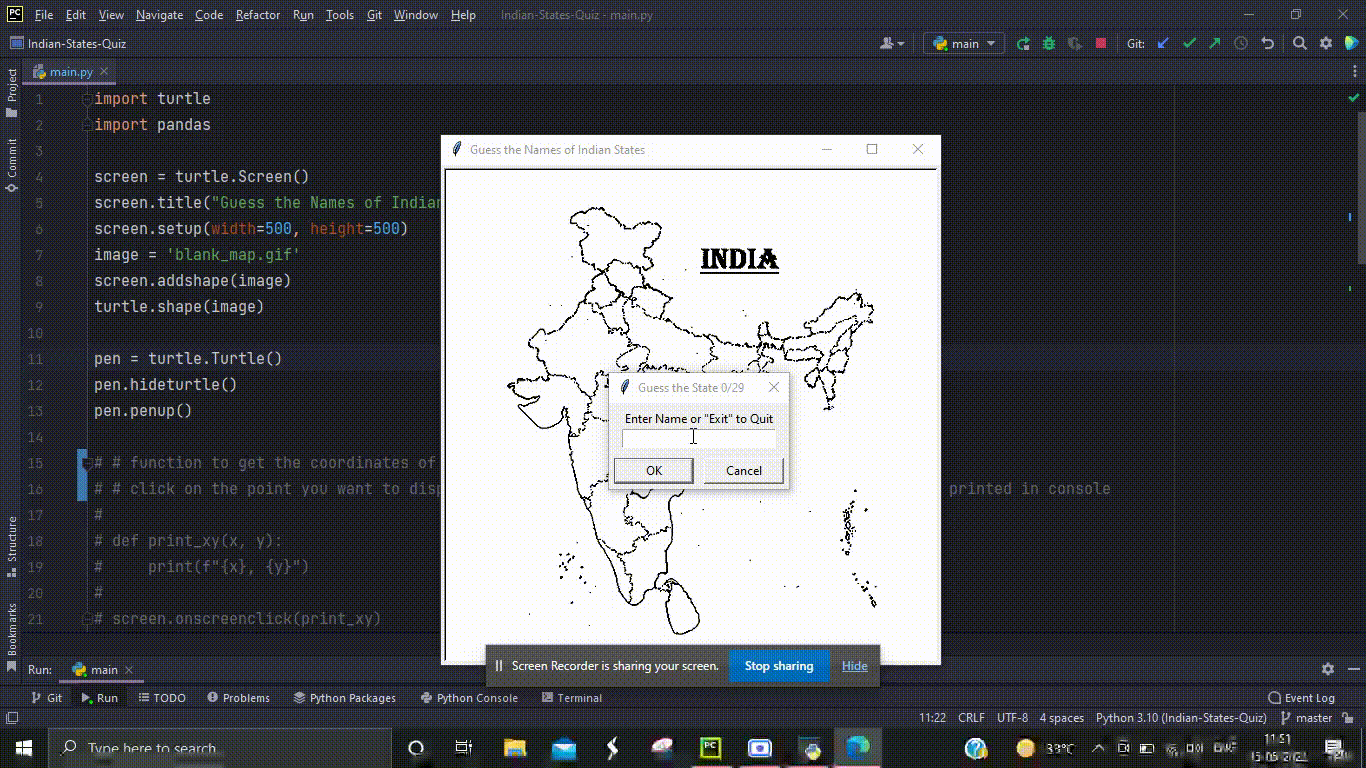Open In App
Related Articles

# Build GUI Application for Guess Indian State using Tkinter Python

In this article, you will learn to make a GUI game in Python, in which we will be shown a map of Indian states and an input prompt were in the prompt, the user has to enter a state name of India. If the user guessed it correctly, the name would hover over its location on the map. You can also see the score as the title of the input prompt window.

## Modules needed

• Python3 – Python is a high-level, general-purpose, and very popular programming language. It is being used in web development, Machine Learning applications, e.t.c.
• Turtle – “Turtle” is a Python feature like a drawing board, which lets us command a turtle to draw all over it
• Pandas – Pandas package offers various data structures and operations for manipulating numerical data and time series.

## Requirements:

• A blank political map of India.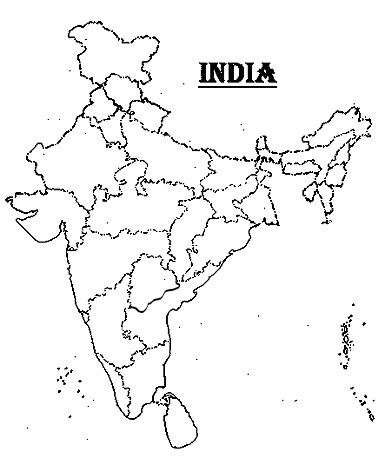• A CSV sheet that will hold data of coordinates.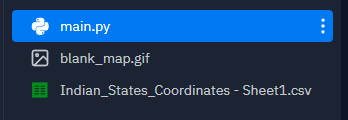## Stepwise Implementation

Step 1: The initial setup

The first step is to import our libraries and create the basic setup, in which a window pop up and we have the map of India covering the screen. We also have a title for our window and a turtle through which we draw or write on the screen. Currently, the turtle doesn’t have to do anything, but it needs to be hidden. The height and width were given according to the size of the map. If the user would like to choose some other map, set the length and width accordingly. Also note that in order to work with images in Turtle, the image must be in GIF format.

## Python3

 `# IMPORTING THE REQUIRED LIBRARIES``import` `turtle``import` `pandas` `# SETTING UP THE WINDOW THAT OPENS``# IN WHICH WE PLAY OUR GAME` `# creating a blank window from Screen()``# class of turtle library``screen ``=` `turtle.Screen()` `# give a title to our window using``# title method of screen object``screen.title(``"Guess the Names of Indian States"``)` `# give the window height and width``# in which our map fits perfectly``screen.setup(width``=``500``, height``=``500``)` `# use addshape method of screen object``# to add an image to our program``image ``=` `'blank_map.gif'``screen.addshape(image)` `# give the turtle the shape of our image``turtle.shape(image)` `# SETTING UP THE TURTLE` `# create a turtle from Turtle class``pen ``=` `turtle.Turtle()` `# we don't want to see it hovering``# over our map, so we hide it``pen.hideturtle()` `# we don't want to draw anything,``# so we lift it up from screen.``# like lifting the pen from paper``# to leave a blank space while writing``pen.penup()` `# this method keep the window on for``# display until the user himself closes it.``# this method should always be the last``# line of our code whenever using turtle.``turtle.mainloop()`

Output: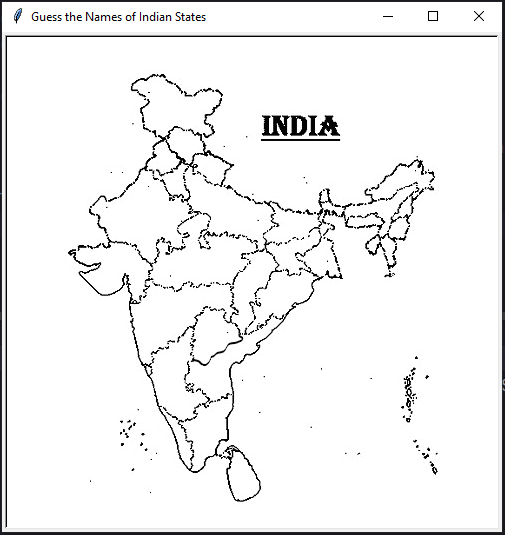Step 2: Creating the data for coordinates of our States.

In this step, we will locate our states once the name is entered. Also, we need coordinates of the states for that, we will use the following code, which prints the x and y coordinates of the point where we click. Remember that the center of the window is the origin of our coordinate system. When you get the coordinates of all states of India printed on the console, copy them and paste them into an excel or google sheet against their respective state names and save it as a CSV file. After saving the file you can comment on the following code.

## Python3

 `# function to get the coordinates of states.``# click on the point you want to display``# state name and then the x and y``# coordinates will be printed in console``def` `print_xy(x, y):``    ``print``(f``"{x}, {y}"``)`  `# onscreenclick is an event listener``# that calls a function everytime we``# click on screen.``screen.onscreenclick(print_xy)`

You can see how it looks when you click on the screen. Now click on points where you would want your state name to be displayed after its name is entered.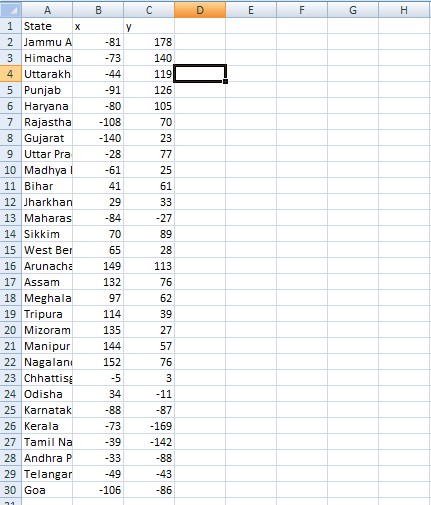Step 3: Get the Data from CSV.

The next step is to create a list of state names that we can use in our program. We read the complete data from the CSV file using the Pandas library and its method, and then extract the values from the ‘State’ column.

## Python3

 `# use read_csv method to read``# entire data from the file``data ``=` `pandas.read_csv(``'Indian_States_Coordinates - Sheet1.csv'``)` `# extract the values under "State"``# column and convert it to a list``states ``=` `data[``'State'``].to_list()` `# make an empty list of correct guesses,``# that we will use to see how many``# correct guesses the user makes while``# playing the game.``correct_guesses ``=` `[]`

Step 4:  Game Play

Here because there are 29 states in India, we shall repeat the loop 29 times. Because the total of our correct guesses list is zero at first, the loop will continue to execute until the list’s length is less than 29. The first step is to create an input prompt to ask the user for his response. He will enter the state name if he wants to play; else, he will enter “Exit” to exit. Convert the user’s answer to the title case, just as all of our state names are, because the user may have entered the answer in a different case. We don’t want to inform him that his answer was incorrect because his situation differed from ours. If the user chooses not to play, the loop is broken after generating a CSV file from the Data Frame with the names of states that the user failed to predict. Then we check to see if the user’s response is correct. We retrieve the state’s data from our data set using that answer, which is the x and y coordinates.

## Python3

 `# we will run the game until our correct``# guesses include all the states``while` `len``(correct_guesses) < ``29``:` `    ``# create an input prompt to ask user``    ``# to enter a state name if he wants to``    ``# exit, he should enter "Exit"``    ``answer ``=` `screen.textinput(``        ``title``=``f``'Guess the State {len(correct_guesses)}/29'``,``                  ``prompt``=``'Enter Name or "Exit" to Quit'``)``    ` `    ``# convert the user to Title case, which``    ``# is the case in which we have stored``    ``# our state names. We need to convert user``    ``# input in case he/she enter the name in``    ``# some other case``    ``answer ``=` `answer.title()` `    ``# first check if the user want to quit.``    ``if` `answer ``=``=` `"Exit"``:``        ``# create a list of state names he missed``        ``# and store them in a dictionary``        ``missing_states ``=` `[n ``for` `n ``in` `states ``if` `n ``not` `in` `correct_guesses]``        ``missing_guess ``=` `{``            ``'State'``: missing_states``        ``}``        ` `        ``# create a dataframe using Pandas method``        ``# and convert and store it as CSV file``        ``pandas.DataFrame(missing_guess).to_csv(``            ``'states_to_learn.csv'``, index``=``False``)``        ``# break the game loop``        ``break` `    ``# check if the answer user entered is in the``    ``# states list or not``    ``if` `answer ``in` `states:``        ``# extract that state's data from our data set``        ``state ``=` `data[data.State ``=``=` `answer]``        ``x_ ``=` `int``(state.x)``        ``y_ ``=` `int``(state.y)``        ` `        ``# add this correct answer to our correct``        ``# guesses list``        ``correct_guesses.append(answer)``        ` `        ``# now go to required coordinates and write``        ``# the state name``        ``pen.goto(x``=``x_, y``=``y_)``        ``pen.write(f``"{answer}"``, font``=``(``'Arial'``, ``8``, ``'normal'``))`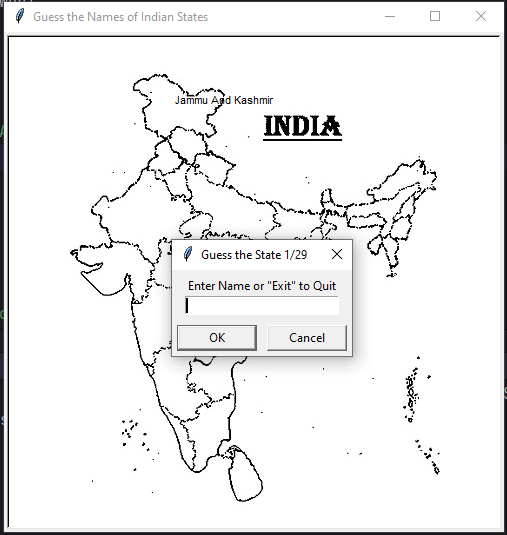Step 5:  Game Result

When the loop terminates, we want to display the user’s score and performance. Based on his score, we determine whether his performance is good, average, or poor. We clear the entire window to make space for the result in a new blank window. We put the result in the center,

## Python3

 `screen.clear()``pen.goto(``0``, ``0``)``end_text ``=` `""``score ``=` `len``(correct_guesses)` `if` `score ``=``=` `29``:``    ``end_text ``=` `'You Guessed Them All Correctly'``elif` `score >``=` `20``:``    ``end_text ``=` `f``"Good Work: {score}/29"``elif` `score >``=` `15``:``    ``end_text ``=` `f``"Average Performance: {score}/29"``else``:``    ``end_text ``=` `f``"Poor Performance: {score}/29"` `pen.write(f``"{end_text}"``, align``=``'center'``, font``=``(``"Arial"``, ``22``, ``'normal'``))` `turtle.mainloop()`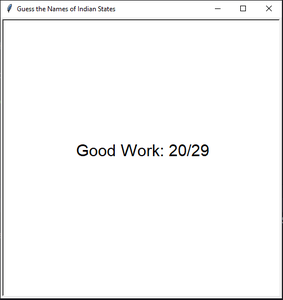## Python3

 `import` `turtle``import` `pandas` `screen ``=` `turtle.Screen()``screen.title(``"Guess the Names of Indian States"``)``screen.setup(width``=``500``, height``=``500``)``image ``=` `'blank_map.gif'``screen.addshape(image)``turtle.shape(image)` `pen ``=` `turtle.Turtle()``pen.hideturtle()``pen.penup()` `# function to get the coordinates of states.``# click on the point you want to display``# state name and then the x and y coordinates``# will be printed in console``data ``=` `pandas.read_csv(``'Indian_States_Coordinates - Sheet1.csv'``)``states ``=` `data[``'State'``].to_list()` `correct_guesses ``=` `[]` `while` `len``(correct_guesses) < ``29``:` `    ``answer ``=` `screen.textinput(``        ``title``=``f``'Guess the State {len(correct_guesses)}/29'``,``      ``prompt``=``'Enter Name or "Exit" to Quit'``)``    ``answer ``=` `answer.title()` `    ``if` `answer ``=``=` `"Exit"``:``        ``missing_states ``=` `[n ``for` `n ``in` `states ``if` `n ``not` `in` `correct_guesses]``        ``missing_guess ``=` `{``            ``'State'``: missing_states``        ``}``        ``pandas.DataFrame(missing_guess).to_csv(``            ``'states_to_learn.csv'``, index``=``False``)``        ``break` `    ``if` `answer ``in` `states:``        ``state ``=` `data[data.State ``=``=` `answer]``        ``x_ ``=` `int``(state.x)``        ``y_ ``=` `int``(state.y)``        ``correct_guesses.append(answer)``        ``pen.goto(x``=``x_, y``=``y_)``        ``pen.write(f``"{answer}"``, font``=``(``'Arial'``, ``8``, ``'normal'``))` `screen.clear()``pen.goto(``0``, ``0``)``end_text ``=` `""``score ``=` `len``(correct_guesses)``if` `score ``=``=` `29``:``    ``end_text ``=` `'You Guessed Them All Correctly'``elif` `score >``=` `20``:``    ``end_text ``=` `f``"Good Work: {score}/29"``elif` `score >``=` `15``:``    ``end_text ``=` `f``"Average Performance: {score}/29"``else``:``    ``end_text ``=` `f``"Poor Performance: {score}/29"` `pen.write(f``"{end_text}"``, align``=``'center'``, font``=``(``"Arial"``, ``22``, ``'normal'``))` `turtle.mainloop()`

Output: